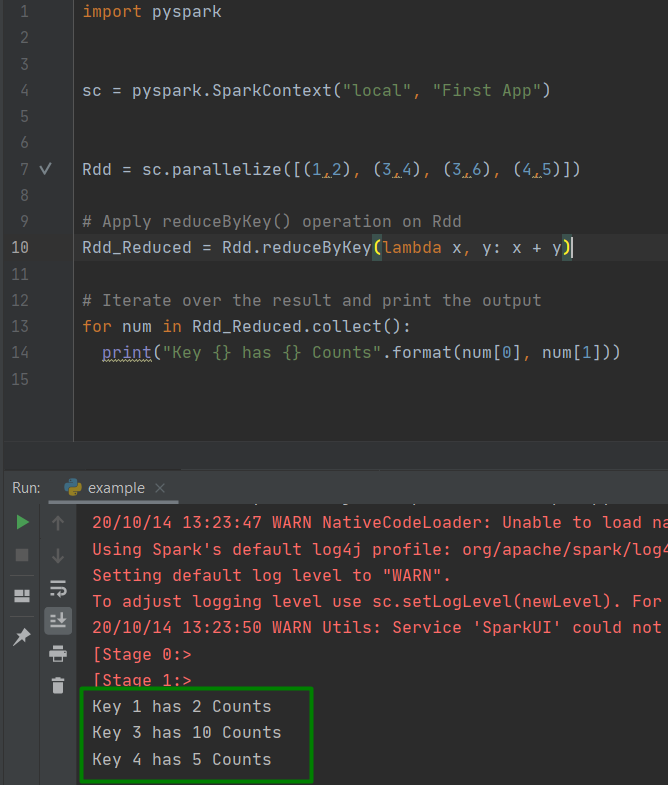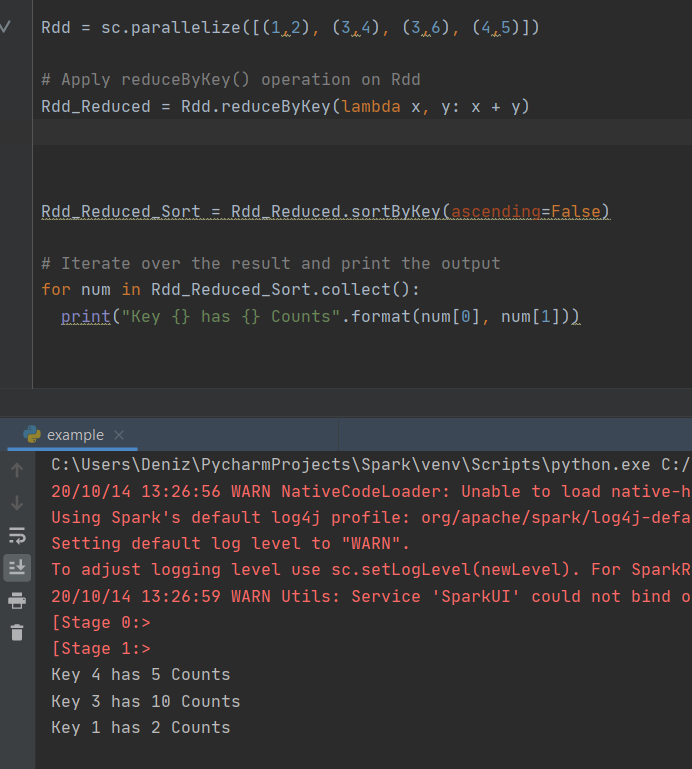# PySpark RDD Example

Hello, in this post we will do 2 short examples, we will use reducebykey and sortbykey.

```Rdd = sc.parallelize([(1,2), (3,4), (3,6), (4,5)])

# Apply reduceByKey() operation on Rdd
Rdd_Reduced = Rdd.reduceByKey(lambda x, y: x + y)

# Iterate over the result and print the output
for num in Rdd_Reduced.collect():
print("Key {} has {} Counts".format(num, num))``````import pyspark

sc = pyspark.SparkContext("local", "First App")

Rdd = sc.parallelize([(1,2), (3,4), (3,6), (4,5)])

# Apply reduceByKey() operation on Rdd
Rdd_Reduced = Rdd.reduceByKey(lambda x, y: x + y)

Rdd_Reduced_Sort = Rdd_Reduced.sortByKey(ascending=False)

# Iterate over the result and print the output
for num in Rdd_Reduced_Sort.collect():
print("Key {} has {} Counts".format(num, num))
```See you in the next article..

8,939 views last month,  2 views today